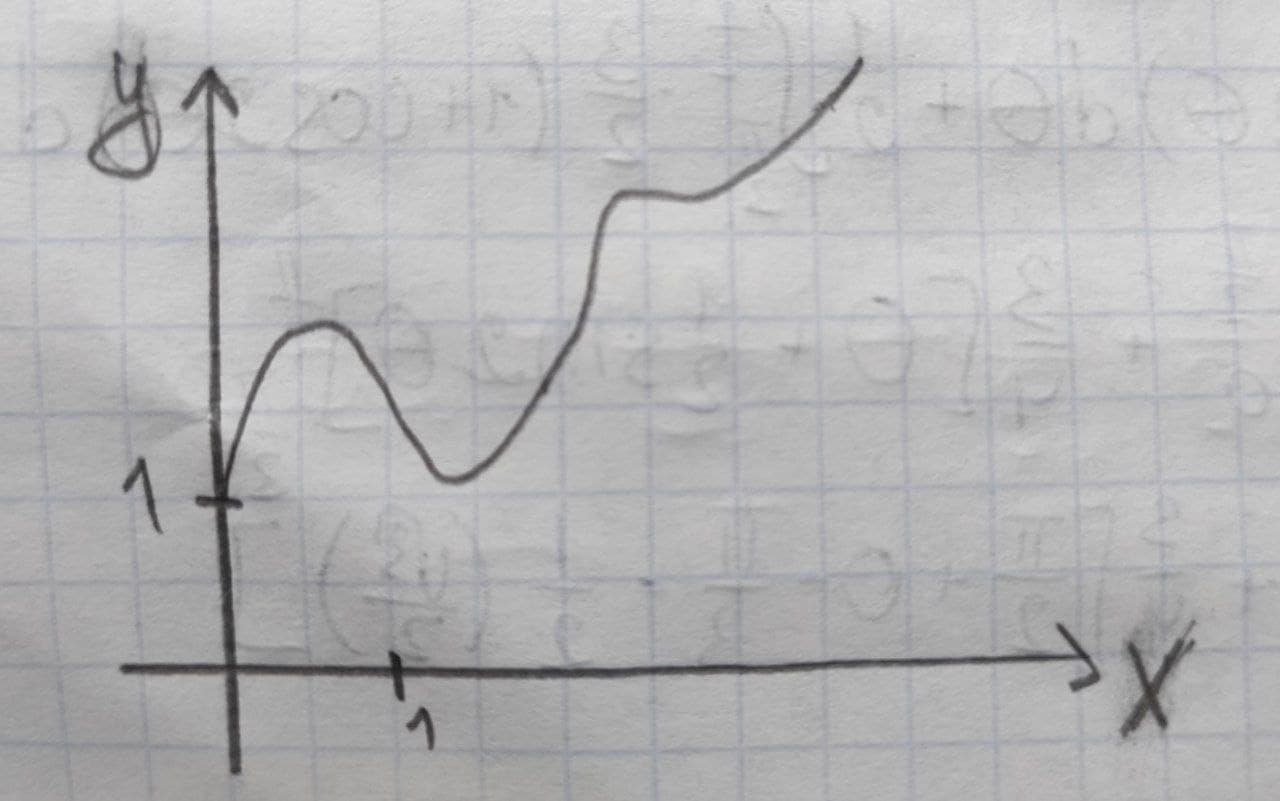Question# Use the given graph of f over the interval (0,7) to find the following.(a) The open intervals on which f is increasing

Parametric equations
ANSWEREDUse the given graph of f over the interval (0,7) to find the following.(a) The open intervals on which f is increasing. (Enter your answer using interval notation.)
(b) The open intervals on which f is decreasing.
(c) The open intervals on which f is concave upward. (Enter your answer using interval notation.)
(d) The open intervals on which f is concave downward. (Enter your answer using interval notation.)
(e) The coordinates of the points of inflection.
(x,y)=( ) (smallest x-value)
(x,y)=( )
(x,y)=( ) (largest x-value)2021-09-11

(a) f is increasing when the graph slopes upwards. In this case, we note that the graph slopes upwards when x is between 0 and 1, when xx is between 3 and 7. Writing this in interval notation, we then obtain
$$\displaystyle{\left({0},{1}\right)}\cup{\left({3},{7}\right)}$$
(b) f is decreasing when the graph slopes downwards. In this case, we note that the graph slopes downwards when x is between 1 and 3. Writing this in interval notation, we then obtain:
(1,3)
(c) f is concave upward, when the function decreases less and less, or the function increase more and more. That is, the slope of the function increases. We note that this occurs when xx is between 2 and 4, and when x is between 5 and 7. Writing this in interval notation, we then obtain:
$$\displaystyle{\left({2},{4}\right)}\cup{\left({5},{7}\right)}$$
(d) f is concave downward, when the function increases less and less, or the function decrease more and more. That is, the slope of the function decreases. We note that this occurs when x is between 0 and 2, and when x is between 4 and 5. Writing this in interval notation, we then obtain:
$$\displaystyle{\left({0},{2}\right)}\cup{\left({4},{5}\right)}$$
(e) The inflection points are the points where the function changes from concave upward to concave downward or from concave downward to concave upward. By part (c) and (d), we note that the inflection points are at x=2, x=4, and x=5. We need to determine the corresponding y-values from the given graph, which then results in the following coordinates of the points of inflection:
$$(x,y)=(2,2)$$
$$(x,y)=(4,3)$$
$$(x,y)=(5,4)$$